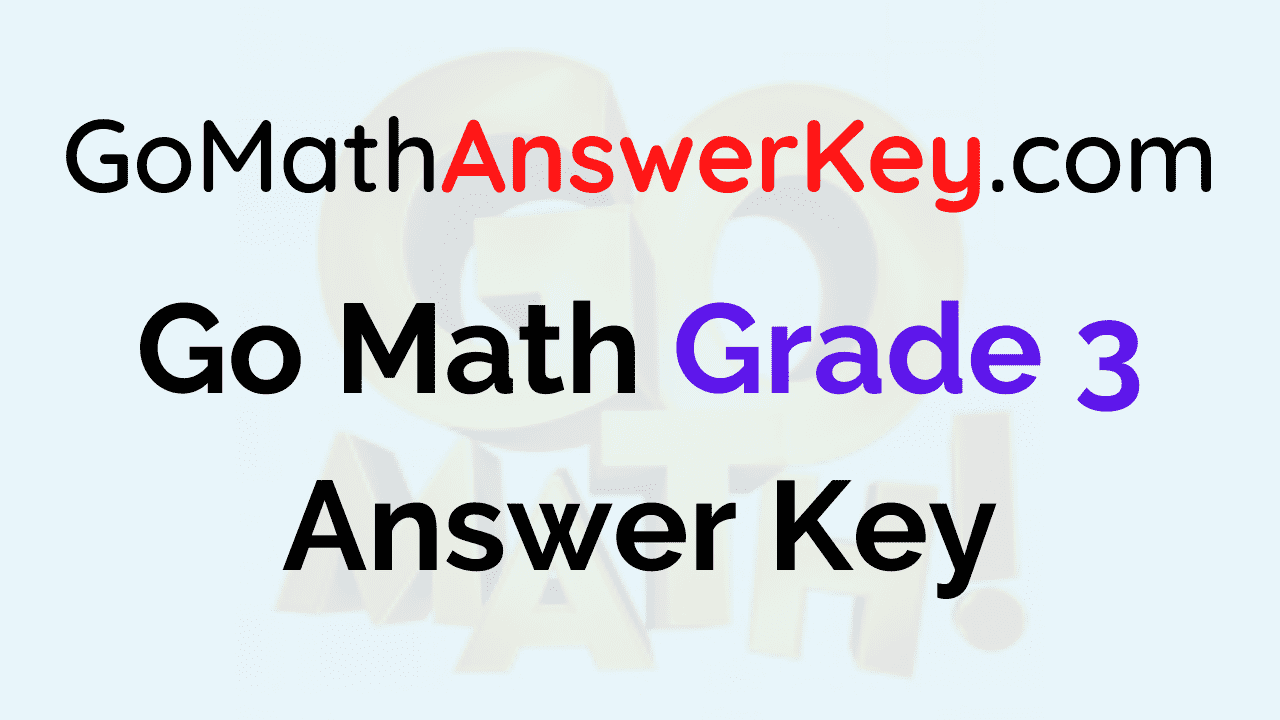Go Math Answer Key for Grade 3: Students of Grade 3 can get a strong foundation on mathematics concepts by referring to the Go Math Course Book. It was developed by highly professional mathematics educators and the solutions prepared by them are in a concise manner for easy grasping. To achieve high scores in Grade 3, students need to solve all questions and exercises included in HMH Go Math Grade 3 Book. Go Math book considers both the content and intent of the Grade 3 school curricula.

So, teachers and students can find this Go Math Answer Key for Grade 3 more helpful in raising students’ scores and supporting teachers to educate the students. By using the HMH Go Math Grade 3 Answer Key, you can easily finish your assessment and can grab good knowledge about all Math chapters. Get step by step explanation of the solution for each question from this Grade 3 Go Math Answer key and master in primary mathematics concepts.

Go Math Answer Key for Grade 3 aids teachers to differentiate instruction, building, and reinforcing foundational mathematics skills that alter from the classroom to real life. With the help of Go Math Primary School Grade 3 Answer Key, you can think deeply regarding what you are learning, and you will really learn math easily just like that. So, download chapterwise Go Math Answer Key Pdf for Grade 3 from the accessible links available over here and make your learning more efficient.

### Grade 3 HMH Go Math – Extra Practice Questions and Answers

• Chapter 1: Addition and Subtraction within 1,000 Assessment Test
• Chapter 2: Represent and Interpret Data Assessment Test
• Chapter 3: Understand Multiplication Assessment Test
• Chapter 4: Multiplication Facts and Strategies Assessment Test
• Chapter 5: Use Multiplication Facts Assessment Test
• Chapter 6: Understand Division Assessment Test
• Chapter 7: Division Facts and Strategies Assessment Test
• Chapter 8: Understand Fractions Assessment Test
• Chapter 9: Compare Fractions Assessment Test
• Chapter 10: Time, Length, Liquid Volume, and Mass Assessment Test
• Chapter 11: Perimeter and Area Assessment Test
• Chapter 12:Two-Dimensional Shapes Assessment Test

Following are some bunch of benefits that students and teachers can avail by referring to the HMH Go Math Solutions Key for Grade 3:

• Teachers can learn new techniques and educate students very well during their mathematics classes.
• GO Math Solution Key provides a seamless way to guarantee that students can access the content at proper levels of depth and rigor.
• An in-depth explanation of solutions helps students to succeed in high-stakes assessments.
• Go Math Grade 3 Answer Key pdf of All Chapters are well illustrated with solved examples and practice questions and support to strengthen basic mathematical concepts.

You can even more get some other benefits too with Go Math Solution Key for Grade 3. Hence, mathematics educators advise students to check out the HMH Go math primary school grade 3 answer key and score better marks. Access the respective chapter link provided here and practice more and more for clarifying all your queries at one go.

### FAQs on Go Math Solution Key for Grade 3

1. How do I solve mathematics questions easily?

Solving math problems easily requires more practice and a strong foundation on primary mathematics concepts. Learning math topics from the Go Math Primary School Solution Key is the smartest way. Even you can easily answer all types of questions during preparation or in the exams.

2. How can I find HMH Go Math Answer Key for Grade 3?

You can easily attain HMH Go math grade 3 Anwer key from our page along with step by step solutions for each question of all chapters.

3. Where can I get the solutions for all chapters Go Math Grade 3 Questions?

Go Math Solution Key is the best guide to get all solved questions and answers as well as extra practice questions. Avail the grade 3 Go Math Answer Key and get a good grip on all primary mathematics concepts.

4. Which is the best website to get free Go Math Answer Key for Grade 3?

Aplustopper.com is a genuine and reliable website that provides Go Math Answer Key for 3rd Grade students. Download 3rd Std HMH Go Math Solution Key pdf for all chapters from the site for free and kick start your preparation and score well in the exams.

Scroll to Top